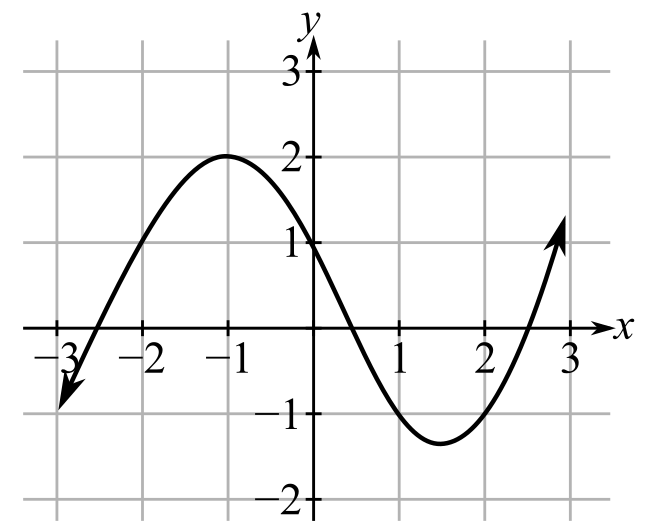Chapter 10.2, Problem 2EFinite Mathematics and Applied Cal...

7th Edition
Stefan Waner + 1 other
ISBN: 9781337274203

Solutions

Chapter
SectionFinite Mathematics and Applied Cal...

7th Edition
Stefan Waner + 1 other
ISBN: 9781337274203
Textbook Problem

In Exercises 1–14 the graph of a function f is given. Determine whether f is continuous on its domain. If it is not continuous on its domain, say why. [HINT: See Quick Examples 1–4.]To determine

Whether the function f is continuous on its domain and give reason if it is not continuous. The graph of function f is as follows:Explanation

Given Information:

The provided graph is:

Consider the provided graph:

Still sussing out bartleby?

Check out a sample textbook solution.

See a sample solution

The Solution to Your Study Problems

Bartleby provides explanations to thousands of textbook problems written by our experts, many with advanced degrees!

Get Started

In Exercises 25-30, find the rules for the composite functions f g and g f. 28. f(x)=2x+3;g(x)=x2+1

Applied Calculus for the Managerial, Life, and Social Sciences: A Brief Approach

In problems 19-22, rewrite the expression with positive exponents. 19.

Mathematical Applications for the Management, Life, and Social Sciences

If p 1. evaluate the expression 1+12P+13P+14P+112P+13P14P+

Single Variable Calculus: Early Transcendentals

True or False: is a convergent series.

Study Guide for Stewart's Single Variable Calculus: Early Transcendentals, 8th